# Triangle - Definition with Examples

The Complete K-5 Math Learning Program Built for Your Child

• 40 Million Kids

Loved by kids and parent worldwide

• 50,000 Schools

Trusted by teachers across schools

• Comprehensive Curriculum

Aligned to Common Core

## Triangle Games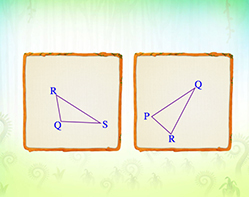Types of Triangles

Classify triangles based on side lengths and angle measures. Look for acute, obtuse and right angles and identify equal sides.

Covers Common Core Curriculum 4.G.2

## What is a Triangle?

In geometry, a triangle is a closed, two-dimensional shape with three straight sides. A triangle is also a polygon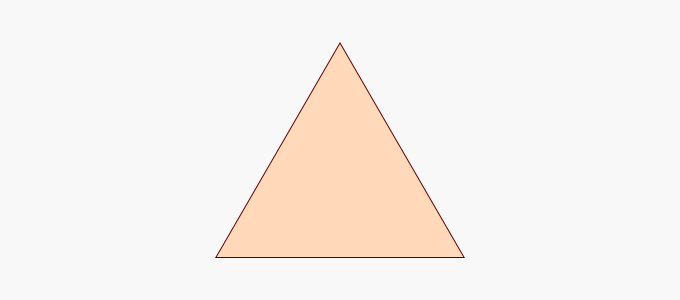We can find the shape of a triangle in a flag, the musical instrument triangle, and a roadside signboard.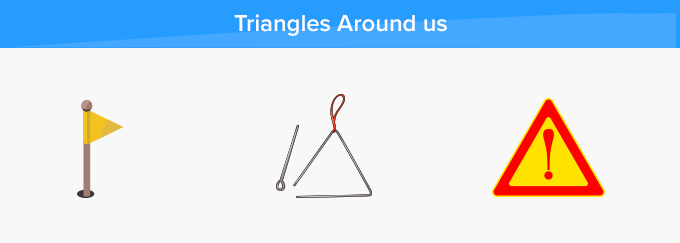## Properties of a Triangle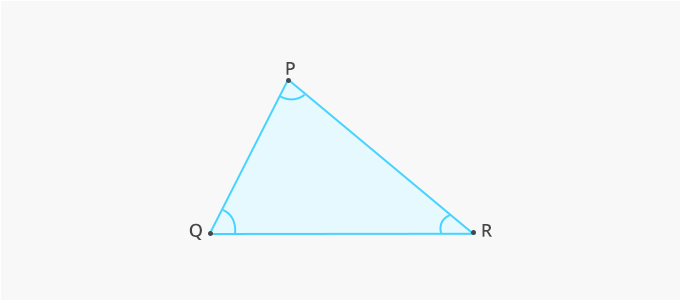• A triangle has three sides, three vertices, and three angles.
• The sum of the three interior angles of a triangle is always 180°.
• The sum of the length of two sides of a triangle is always greater than the length of the third side.
• A triangle with vertices P, Q, and R is denoted as △PQR.
• The area of a triangle is equal to half of the product of its base and height.

## Different Types of Triangles

To classify triangles according to their angles, we measure each of its interior angles. Triangles can be classified by angles, as:

 Acute Triangle Right Triangle Obtuse Triangle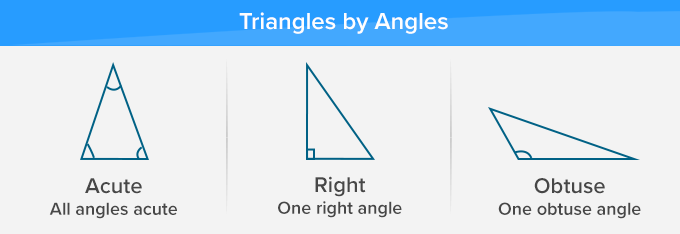An acute triangle has all interior angles acute (less than 90°), a right triangle has one right angle (equal to 90°) and an obtuse triangle has one obtuse angle (greater than 90°).

To classify the triangles according to their sides, we measure the length of each of its sides. Triangles can be classified by their sides, as:

 Equilateral Triangle Isosceles Triangle Scalene Triangle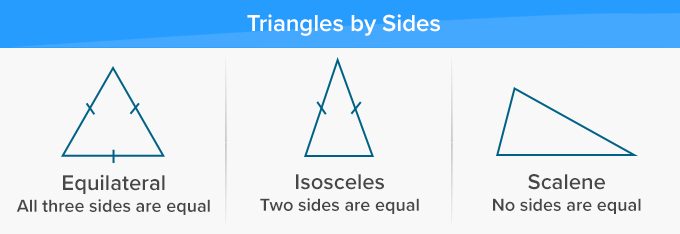To classify triangles according to both angles and sides, we measure the interior angles and length of the sides of the triangle. Few examples of triangles classified on the basis of both angles and sides are:

 Acute Equilateral Triangle Right Isosceles Triangle Obtuse Scalene Triangle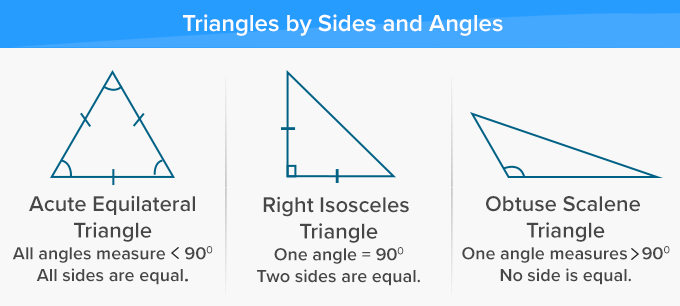Fun Facts A triangle can always be split into two right triangles, irrespective of its orientation. Triangles are polygons with the least number of sides.

##Let's sing!

Jenna loves triangles!
Tiny triangles on her blouse,
The red roof of her dollhouse,
A piece of cheese for her mouse.

##Related math vocabulary-